2018-07-17 22:34:21 BAR_WORKSHOP 阅读数 6044
• ###### OpenGL视频教程

初级教程共11个课时，在教程中，我们将从基本的开始，你将学会如何创建窗口，然后，会学到如何创建一个三维的网格？ 你会学到如何使用强大的着色器进行编程，如何创建纹理，如何使用光照， 终实现一套完整的渲染管线，得到一个猴子的模型。

56920 人正在学习 去看看 黄棒清

1.绘图工具箱

 plot 基本绘图函数 mesh 绘制三维网格曲面 fplot 绘制数学函数图 surf 绘制着色的三维彩色曲面 ezplot 直接绘制隐函数图形 meshgrid 针对三维图形的x和y数组 bar 绘制条形图 semilogx 绘制x对数坐标系图 area 绘制面积图 semilogy 绘制y对数坐标系图 pie 绘制饼形图 loglog 绘制对数坐标系图 title 标题 contour 绘制等高线图 hist 绘制直方图 stem 绘制火柴杆图 axis 控制轴的比例和外观 grid 网格线 xlabel x-轴标记 ylabel y-轴标记

2.图像的显示

 imshow 以处理图像的方式显示图像 imtool 以图像工具的方式显示图像 subimage 以单幅图像的形式显示排列好的多幅图像 immovie 多帧图像制成电影 implay 播放电影，视频或图像序列 montage 矩形蒙太奇显示多幅图像 warp 纹理映射表面

3.几何变换

 imrotate 旋转图像 makeresampler 创建重取样结构 imcrop 裁剪图象 maketform 创建空间变换结构（TFORM） imresize 调整图像大小 fliptform 转换TFORM结构的输入和输出角色 checkerboard 创建棋盘格图像 tformarray 对N-D阵列施以空间变换 findbounds 为空间变换寻找输出边界 tformfwd 应用正向空间变换 imtransform 对图像进行二维空间变换 tforminv 应用逆向空间变换 impyramid 以金字塔形式缩减或扩展图像

4.像素值和统计

 imhist 显示图像数据的直方图 std2 矩阵元素的标准差 impixel 像素彩色值 mean2 矩阵元素的平均或均值 imcontour 创建图像数据轮廓线 corr2 二维相关系数 improfile 沿着线段的横截面的像素值 regionprops 度量图像区域的特性

5.图像变换

 fanbeam 扇形射束变换 fan2para 把扇形射束投影变换为平行射束 ifanbeam 反扇形射束变换 para2fan 把平行射束投影变换为扇形射束 radon 雷登变换 dct2 二维离散余弦变换 iradon 反雷登变换 idct2 二维反离散余弦变换 phantom 创建头部幻影图像 dctmtx 离散余弦变换矩阵

6.图像分析

 bwtraceboundary 追踪二值图像中的目标 qtdecomp 四叉树分解 edge 寻找灰度图像的边缘 qtgetblk 得到块值 hough 霍夫变换 qtsetblk 设置块值 houghlines 基于霍夫变换的线段提取 houghpeaks 识别霍夫变换的峰

7.图像算术

 imabsdiff 计算两幅图像的绝对差 imlincomb 图像的线性组合 imcomplement 图像求补 ippl 检查IPPL是否存在

8.图像去模糊

 deconvblind 盲去卷积法 edgetaper 点扩散函数渐变边缘 deconvlucy Lucy-Richardson方法 otf2psf 把光传递函数转换为点扩散函数 deconvreg 规则滤波器法 psf2otf 把点扩散函数转换为光传递函数 deconvwnr 维纳滤波器法

9.线性滤波以及线性二维滤波器设计

 convmtx2 二维卷积矩阵 fsamp2 频率取样的二维FIR滤波器 fspecial 创建预定义的二维滤波器 ftrans2 频率变换的二维FIR滤波器 imfilter 多维图像的N-D滤波 fwind1 一维窗方法的二维FIR滤波器 freqz2 二维频率响应 fwind2 二维窗方法的二维FIR滤波器

10.图像配准

 normxcorr2 归一化的二维互相关 cp2tform 用控制点对推断空间变换 cpselect 控制点选择工具 cpstruct2pairs 把CPSTRUCT转换为控制点对 cpcorr 用互相关调整控制点位置

11.图像增强

 histeq 用直方图均衡增强对比度 wiener2 二维自适应噪声去除滤波 imadjust 调整图像亮度值或彩色图 intlut 用查表法转换整数值 medfilt2 二维中值滤波 adapthisteq 有限对比度的自适应直方图均衡CLAHE ordfilt2 二维统计排序滤波 stretchlim 寻找如何限制一幅图像的对比度拉伸

12.形态学操作

 灰度图像和二值图像 二值图像 conndef 默认的连通性数组 imhmax 最大H变换 imbothat 底帽滤波 imhmin 最小H变换 imclearborder 连接到图像边缘的抑制光结构 imimposemin 强迫最小 mclose 形态学闭操作图像 imopen 形态学开操作图象 imdilate 膨胀图像 imreconstruct 形态学重建 imerode 腐蚀图像 imregionalmax 区域最大 imextendedmax 最大扩展变换 imregionalmin 区域最小 imextendedmin 最小扩展变换 imtophat 顶帽滤波 imfill 填充图像区域和孔洞 watershed 分水岭变换 applylut 查表法的领域操作 bwmorph 二值图像的形态学操作 bwarea 二值图像中的目标区域 bwpack 打包二值图像 bwareaopen 形态学开二值图像 bwperim 寻找目标的周长 bwdist 二值图像的距离变换 bwselect 选择目标 bweuler 二值图像的欧拉数 bwulterode 最终腐蚀 bwhitmiss 二元击中-击不中操作 bwunpack 拆包二值图像 bwlabel 二维二值图像中标记连通分量 makelut 为了使用APPLYLUT而创建查找表 bwlabeln N维二值图像中标记连通分量

13.彩色空间

 cmpermute 在彩色图中重新安排彩色 lab2uint8 L*a*b转变为uint8 cmunique 去除索引图像的彩色图中不需要的颜色 xyz2double xyz颜色值转变为double imapprox 用较少颜色之一近似索引图像 xyz2uint16 xyz颜色值转变为uint16 ntsc2rgb NTSC转变为RGB makecform 创建独立于设备的彩色空间变换结构CFORM ycbcr2rgb YCbCr转变为RGB applyform 适用于与设备无关的彩色空间变换 rgb2ntsc RGB转变为NTSC iccfind 搜索ICC剖面 rgb2ycbcr RGB转变为YCbCr iccroot 寻找系统的ICC剖面存放处 whitepoint 标准照明的XYZ颜色值 iccwrite 写ICC彩色剖面 lab2double L*a*b转变为double isicc 对完全剖面结构为真 lab2uint6 L*a*b转变为uint16

14.邻域和块处理

 bestblk 块处理的最佳尺寸 col2im 把矩阵列重排为块 blkproc 图像的不同块处理 colfilt 列方式的邻域操作 nlfilter 一般的滑动邻域处理 im2col 把图像块重排为列

2018-12-12 17:03:01 lengjiayi 阅读数 2285
• ###### OpenGL视频教程

初级教程共11个课时，在教程中，我们将从基本的开始，你将学会如何创建窗口，然后，会学到如何创建一个三维的网格？ 你会学到如何使用强大的着色器进行编程，如何创建纹理，如何使用光照， 终实现一套完整的渲染管线，得到一个猴子的模型。

56920 人正在学习 去看看 黄棒清

# 一些基本数字图像处理算法

## 1 图像扭曲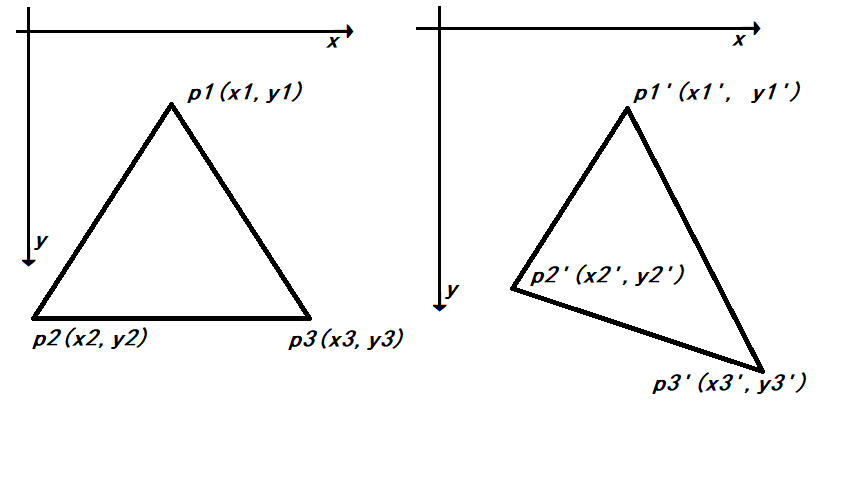$A = \begin{bmatrix} x_{1} & y_{1} & 1 \\ x_{2} & y_{2} & 1 \\ x_{3} & y_{3} & 1 \\ \end{bmatrix}$

B为形变后顶点的其次矩阵：

$B = \lbrack\begin{matrix} x_{1}^{'} & x_{2}^{'} & x_{3}^{'} \\ y_{1}^{'} & y_{2}^{'} & y_{3}^{'} \\ \end{matrix}\rbrack$

M即为$2 \times 3$的映射矩阵，且由于三角形三点不共线，因此A为可逆阵，

$M = BA^{- 1}$

## 3 直方图均衡化算法

$D_{B} = f\left( D_{A} \right),\ H_{B}\left( D_{B} \right)\Delta D_{B} = H_{A}\left( D_{A} \right)\Delta D_{A}$

$H_{B}\left( D_{B} \right) = \frac{H_{A}\left( D_{A} \right)\Delta D_{A}}{\Delta D_{B}} = \frac{H_{A}\left( D_{A} \right)}{\frac{\Delta D_{B}}{\Delta D_{A}}} = \frac{H_{A}\left( D_{A} \right)}{\frac{dD_{B}}{dD_{A}}}$

$= \frac{H_{A}\left( D_{A} \right)}{f'(D_{A})} = \frac{H_{A}\left( f^{- 1}\left( D_{B} \right) \right)}{f'(f^{- 1}(D_{B}))}$

$f\left( D \right) = D_{m}CDF(D)$，CDF即累积分布函数

## 5 图像二值化

$g = w_{0}w_{1}\left( u_{0} - u_{1} \right)^{2}$

## 7 滤波

$\begin{bmatrix} 1 & 2 & 3 & 2 & 1 \\ 2 & 4 & 6 & 4 & 2 \\ 3 & 6 & 7 & 6 & 3 \\ 2 & 4 & 6 & 4 & 2 \\ 1 & 2 & 3 & 2 & 1 \\ \end{bmatrix}$

## 8 形态学扩张和腐蚀

$f\Theta b\left( x,y \right) = min\{ f\left( x - x^{'},\ y - y^{'} \right) - b(x^{'},y')|(x^{'},y^{'} \in D_{b})\}$

$f\bigoplus b\left( x,y \right) = max\{ f\left( x - x^{'},\ y - y^{'} \right) - b(x^{'},y')|(x^{'},y^{'} \in D_{b})\}$

## 9 傅里叶变换和滤波

### 变换公式

$F\left( u,v \right) = \iint_{}^{}{f\left( x,y \right)e^{- 2\pi\overrightarrow{j}(ux + vy)}}\text{dxdy}$

$f\left( x,y \right) = \iint_{}^{}{F\left( u,v \right)e^{2\pi\overrightarrow{j}(ux + vy)}}\text{dudv}$

$G\left( m,n \right) = \frac{1}{\sqrt{\text{MN}}}\sum_{\begin{matrix} 0 \leq \ i\ \leq \ M - 1 \\ 0 < k < N - 1\ \\ \end{matrix}}^{}{g\left( i,k \right)e^{- 2\pi\overrightarrow{j}(\frac{\text{im}}{M} + \frac{\text{jn}}{N})}}$

$g\left( i,k \right) = \frac{1}{\sqrt{\text{MN}}}\sum_{\begin{matrix} 0 \leq \ m\ \leq \ M - 1 \\ 0 < n < N - 1\ \\ \end{matrix}}^{}{g\left( m,n \right)e^{2\pi\overrightarrow{j}(\frac{\text{im}}{M} + \frac{\text{jn}}{N})}}$

DFT可以理解为对连续二维信号进行了频率为M,
N的采样，之后通过计算其和频域空间M*N个基向量的相关性（在该方向投影）将时域信号映射到频域。iDFT可以理解为通过M*N个基向量合成原始时域信号。

### 算法实现

$G = \mathcal{W}^{*}g\mathcal{W =}\left( \mathcal{W}_{\text{re}} - \overrightarrow{j}\mathcal{W}_{\text{lm}} \right)g\left( \mathcal{W}_{\text{re}} + \overrightarrow{j}\mathcal{W}_{\text{lm}} \right) = \mathcal{W}_{\text{re}}g\mathcal{W}_{\text{re}} + \mathcal{W}_{\text{lm}}g\mathcal{W}_{\text{lm}} - \overrightarrow{j}\left( \mathcal{W}_{\text{lm}}g\mathcal{W}_{\text{re}} + \mathcal{W}_{\text{re}}g\mathcal{W}_{\text{lm}} \right)$

$\left\{ \begin{matrix} G_{\text{re}} = \mathcal{W}_{\text{re}}g\mathcal{W}_{\text{re}} + \mathcal{W}_{\text{lm}}g\mathcal{W}_{\text{lm}} \\ G_{\text{lm}} = - \mathcal{W}_{\text{lm}}g\mathcal{W}_{\text{re}} - \mathcal{W}_{\text{re}}g\mathcal{W}_{\text{lm}} \\ \end{matrix} \right.\$

$g = \left( \mathcal{W}_{\text{re}} + \overrightarrow{j}\mathcal{W}_{\text{lm}} \right)(G_{\text{re}} + {\overrightarrow{j}G}_{\text{lm}})\left( \mathcal{W}_{\text{re}} - \overrightarrow{j}\mathcal{W}_{\text{lm}} \right)$

2019-09-21 17:35:42 d__yz 阅读数 21
• ###### OpenGL视频教程

初级教程共11个课时，在教程中，我们将从基本的开始，你将学会如何创建窗口，然后，会学到如何创建一个三维的网格？ 你会学到如何使用强大的着色器进行编程，如何创建纹理，如何使用光照， 终实现一套完整的渲染管线，得到一个猴子的模型。

56920 人正在学习 去看看 黄棒清

# 什么是数字图像

	数字图像：一个被抽样和量化后的 二维函数（光学方法产生），采用等距矩形网格抽样，对幅度进行等间隔量化


# 图像的分类

	按颜色和灰度的多少可以将图像分为二值图像、灰度图像、索引图像、真彩色RGB图像
大多数图像处理软件都支持这4钟类型的图像


## 二值图像

	仅有0、1两个值构成的二维矩阵，0表示黑色，1表示白色。
数据类型：二进制位
用途：文字、线条图的扫描识别（OCR）和掩膜图像的存储


## 灰度图像

	矩阵取值范围为[0,255]，0表示纯黑色，255表示纯白色，中间值为纯黑色到纯白色 的过渡色
数据类型：八位无符号整形
也可以取值[0,1]，二值图像是特殊的灰度图像


## 索引图像

	由图像的二维矩阵+颜色的索引矩阵MAP构成
图像的二维矩阵存放灰度值，而索引矩阵MAP存放RGB颜色，每一行的二维矩阵的灰度值对应着MAP矩阵的颜色，通过改变索引矩阵即MAP，颜色的形式可以调整的
**图像在屏幕上显示时，每一像素的颜色由存放在矩阵中像素的灰度值作为索引通过检索颜色索引矩阵MAP得到**
数据类型：八位无符号整形
用途：存放色彩要求比较简单的图像


## 真彩色RGB图像

	RGB图像每一个像素的颜色值直接存放在图像矩阵中
3个MxN的二维矩阵分别存放着R、G、B三个颜色分量
数据类型：8位无符号的整形
用途：存放真彩色图像、灰度图像


# 图像文件格式

BMP格式：几乎不进行压缩，占用存储空间大，能被多种Windows应用程序所支持
GIF格式：用来交换图片的，压缩比高，存储空间占用少、不能存储超过256色的图像
JPEG格式：扩展名为.jpg或.jpeg，有损压缩方式去除冗余的图像和彩色数据，具有调节图像质量的功能，用于web浏览图像
JPEG2000格式：具有高压缩率和更多新功能的静态影像压缩技术，支持有损和无损压缩，实现渐进传输（先传轮廓，后传数据），用于扫描仪，数码相机等
TIFF格式：Mac中广泛使用的图像格式，图像格式复杂、存储信息多，有利于原稿的复制，压缩采用LZW无损压缩方案存储，兼容性差
PNG格式：网络图像格式，不失真格式，存储形式丰富，兼有GIF和JPEG的色彩模式，能把图像文件压缩到极限以利于网络传输但还保留所有与图像品质有关的信息，显示速度快，支持透明图像（用网页本身的颜色来代替设为透明的色彩）的制作，不支持动画应用效

2019-04-14 08:16:21 Dujing2019 阅读数 321
• ###### OpenGL视频教程

初级教程共11个课时，在教程中，我们将从基本的开始，你将学会如何创建窗口，然后，会学到如何创建一个三维的网格？ 你会学到如何使用强大的着色器进行编程，如何创建纹理，如何使用光照， 终实现一套完整的渲染管线，得到一个猴子的模型。

56920 人正在学习 去看看 黄棒清

# 数字图像处理—频域处理

## （六）高通(锐化)频域滤波器

1. 增强图像边缘，使模糊的图像变得更加清晰，颜色变得鲜明突出，图像的质量有所改善，产生更适合人眼观察和识别的图像；
2. 希望通过锐化处理后，目标物体的边缘鲜明，以便于提取目标的边缘、对图像进行分割、目标区域识别、区域形状提取等，进一步的图像理解与分析奠定基础。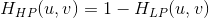#### 6.1 高通滤波函数

function H = hpfilter(typef M, N, DO, n)
if nargin 4 == 4
n = 1;
end
Hlp = lpfilter(type, M, N,DO, n);
H = 1 - Hlp;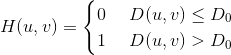H = fftshift(hpfilter('ideal', 500, 500, 50));   %对图像频谱进行移动，使0频率点在中心
figure;
subplot(1, 2, 1), surf(double(H(1:10:500, 1:10:500))), title('理想高通滤波器绘图');
subplot(1, 2, 2), imshow(H,[]), title('理想高通滤波器图像');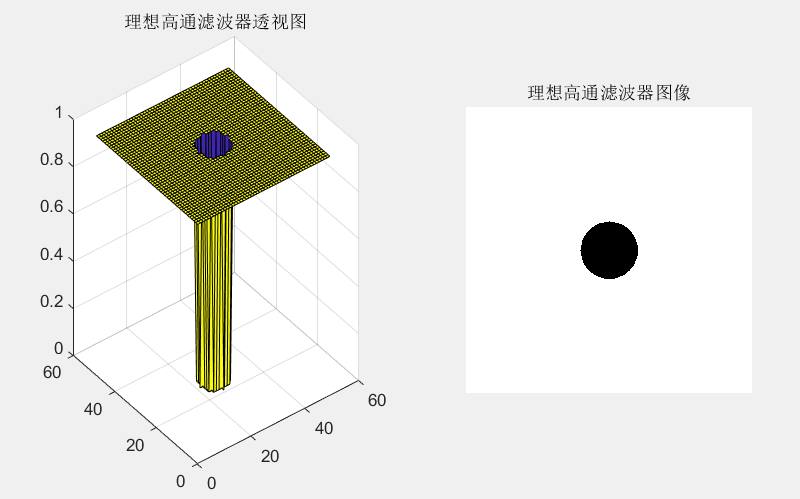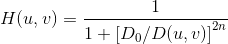H = fftshift(hpfilter('btw', 500, 500, 50));
figure;
subplot(1, 2, 1), surf(double(H(1:10:500, 1:10:500))), title('布特沃斯高通滤波器透视图');
subplot(1, 2, 2), imshow(H,[]), title('布特沃斯高通滤波器图像');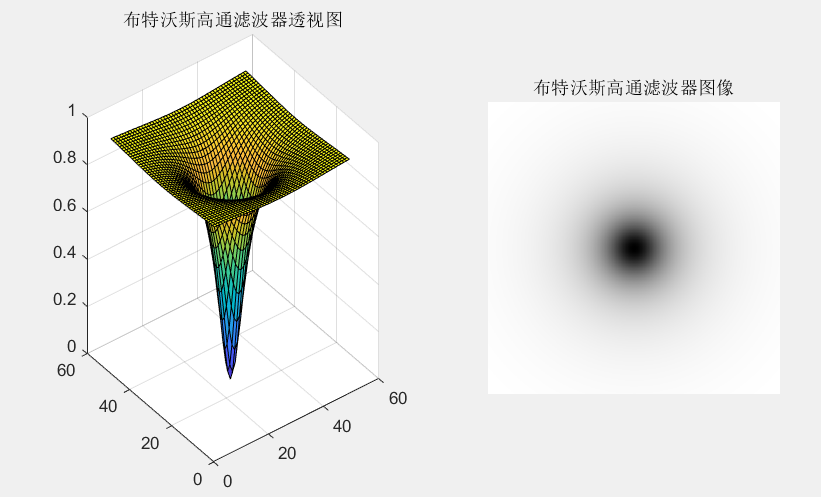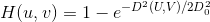H = fftshift(hpfilter('gaussian', 500, 500, 50));
figure;
subplot(1, 2, 1), surf(double(H(1:10:500, 1:10:500))), title('高斯高通滤波器透视图');
subplot(1, 2, 2), imshow(H,[]), title('高斯高通滤波器图像');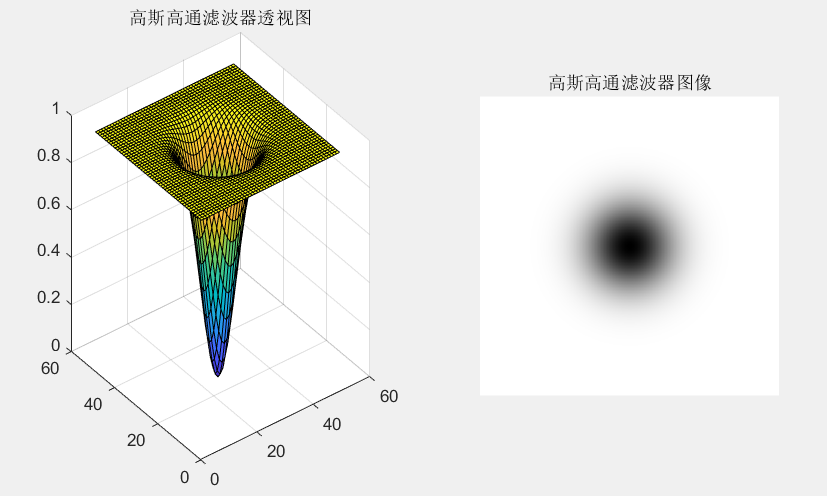w = imread('D:\数字图像处理\第三章学习\blackhole.png');
f = im2double(rgb2gray(w));    %把图像变为灰度图像
[U,V] = dftuv(PQ(1),PQ(2));
DO = 0.05*PQ(2);               %设定滤波器的阈值
F = fft2(f,PQ(1),PQ(2));       %傅里叶变换
H = hpfilter('ideal', PQ(1),PQ(2), DO, 2);   %理想高通滤波
g = dftfilt(f,H);              %用滤波器对图像进行频域滤波
subplot(2, 2, 1), imshow(w), title('原图像');
subplot(2, 2, 2), imshow(fftshift(H),[]), title('以图像显示的理想高通滤波器');
subplot(2, 2, 3), imshow(log(1+abs(fftshift(F))),[]), title('对数增强并居中的fft');
subplot(2, 2, 4), imshow(g,[]), title('理想高通滤波后图像的谱');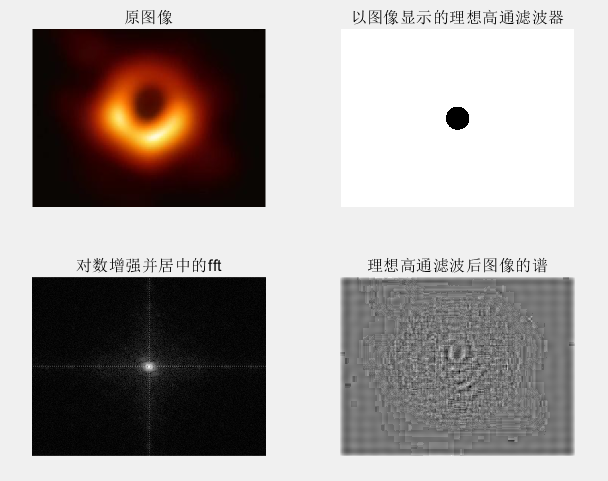w = imread('D:\数字图像处理\第三章学习\blackhole.png');
f = im2double(rgb2gray(w));    %把图像变为灰度图像
DO = 0.05*PQ(1);               %设定滤波器的阈值
H = fftshift(hpfilter('btw', PQ(1),PQ(2), DO));
g = dftfilt(f,H);
figure;
subplot(1, 3, 1), imshow(w), title('原图像');
subplot(1, 3, 2), imshow(f), title('图像的灰度图像');
subplot(1, 3, 3), imshow(g), title('理想高通滤波后结果');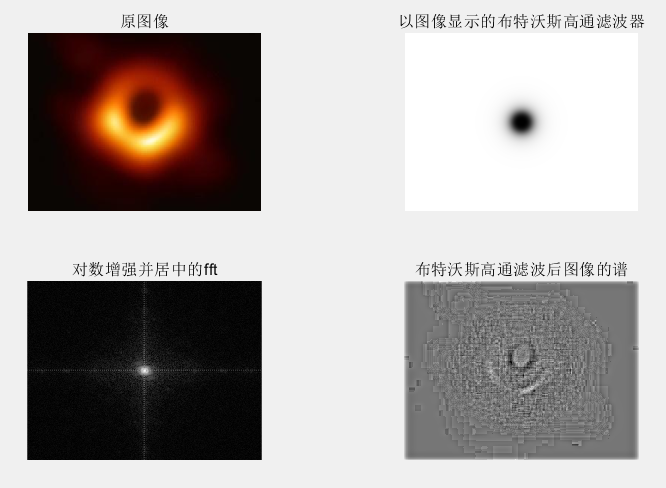w = imread('D:\数字图像处理\第三章学习\blackhole.png');
f = im2double(rgb2gray(w));    %把图像变为灰度图像
[U,V] = dftuv(PQ(1),PQ(2));
DO = 0.05*PQ(2);               %设定滤波器的阈值
F = fft2(f,PQ(1),PQ(2));       %傅里叶变换
H = hpfilter('gaussian', PQ(1),PQ(2), DO, 2);
g = dftfilt(f,H);              %用滤波器对图像进行频域滤波
subplot(2, 2, 1), imshow(w), title('原图像');
subplot(2, 2, 2), imshow(fftshift(H),[]), title('以图像显示的高斯高通滤波器');
subplot(2, 2, 3), imshow(log(1+abs(fftshift(F))),[]), title('对数增强并居中的fft');
subplot(2, 2, 4), imshow(g,[]), title('高斯高通滤波后图像的谱');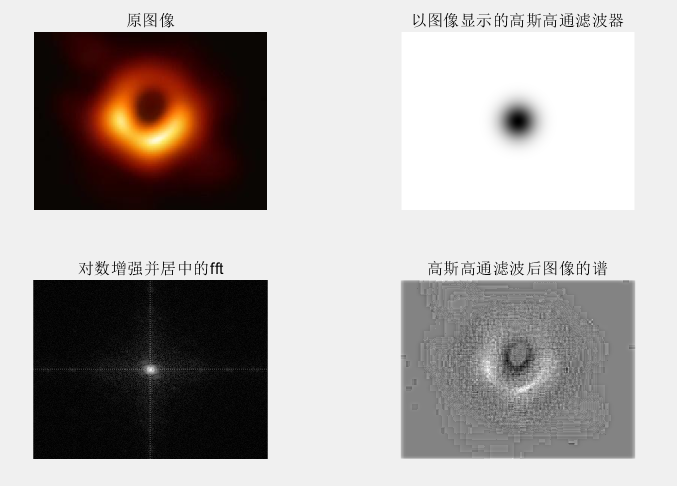#### 6.2 高频强调滤波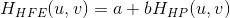a是偏移量，b 是乘数。

w = imread('D:\数字图像处理\第三章学习\blackhole.png');
f = im2double(rgb2gray(w));    %把图像变为灰度图像
DO = 0.05*PQ(1);    %，Do的值等于填充过 的图像垂直尺寸的5%
HBW = hpfilter('btw', PQ(1),PQ(2), DO, 2);
H = 0.5 + 2*HBW;
gbw = dftfilt(f, HBW, 'fltpoint');       %函数 dftfilt 中用 fltpoint 选项以得到浮点结果
gbw = gscale(gbw);
ghf = dftfilt(f, H, 'fltpoint');
ghf = gscale(ghf);
ghe = histeq(ghf, 256);
subplot(2, 2, 1), imshow(w), title('原图像');
subplot(2, 2, 2), imshow(gbw,[]), title('布特沃斯滤波器滤波后的结果');
subplot(2, 2, 3), imshow(ghf,[]), title('高频强调的结果');
subplot(2, 2, 4), imshow(ghe,[]), title('高频强调后经直方图均衡化结果');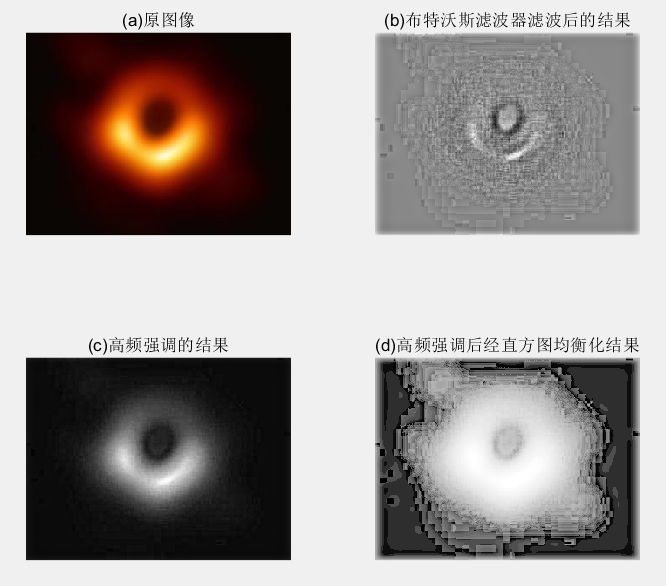## （七）选择性滤波

#### 7.1带阻和带通滤波器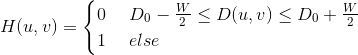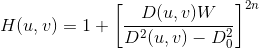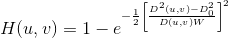#### 7.2 陷波带阻和陷波带通滤波器

w = imread('D:\数字图像处理\第三章学习\blackhole.png');
f = im2double(rgb2gray(w));       %把图像变为灰度图像
[M N] = size(f);
[f,revertclass] = tofloat(f);     %存储输入的类，以便以后使用。
F = fft2(f);                      %傅里叶变换
S = gscale(log(1+abs(fftshift(F))));
C1 = [99 154;128 163];
H1 = cnotch('gaussian','reject', M,N,C1,5);
P1 = gscale(fftshift(H1).*(tofloat(S)));
g1 = dftfilt(f, H1);   %采用默认值 0 填充边缘，频率域滤波函数，第一个参数为原始图像，后者为二维频率域滤器
figure;
subplot(2, 2, 1), imshow(g), title('原图像');
g1 = revertclass(g1);
C2 = [99 154;128 163;49 160;133 233;55 132;108 255;112 74];
H2 = cnotch('gaussian','reject',M,N,C2,5);
P2 = gscale(fftshift(H2).*(tofloat(S)));      %用gscale来进行将数据缩放到默认值0~255
g2 = dftfilt(f,H2);
g2 = revertclass(g2);          %revertclass 可以用于把输出转换回与 f 相同的类。
subplot(2, 3, 1), imshow(w), title('原图像');
subplot(2, 3, 2), imshow(S), title('谱');
subplot(2, 3, 3), imshow(P1), title('应用于由波纹模式导致的低频脉冲的陷波滤波器');
subplot(2, 3, 4), imshow(g1), title('滤波结果');
subplot(2, 3, 5), imshow(P2), title('用更多的滤波器消除高频“结构”噪声');
subplot(2, 3, 6), imshow(g2), title('滤波结果');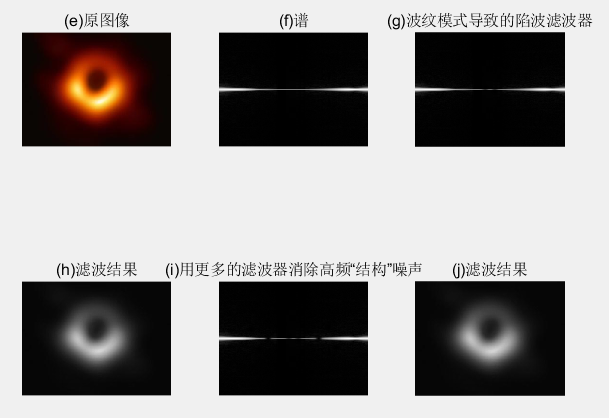2010-09-05 21:32:00 zhywsh 阅读数 1061
• ###### OpenGL视频教程

初级教程共11个课时，在教程中，我们将从基本的开始，你将学会如何创建窗口，然后，会学到如何创建一个三维的网格？ 你会学到如何使用强大的着色器进行编程，如何创建纹理，如何使用光照， 终实现一套完整的渲染管线，得到一个猴子的模型。

56920 人正在学习 去看看 黄棒清

Matlab常用图像操作

. 读写图像文件

2. imwrite

imwrite函数用于写入图像文件，如：imwrite(a,'e:/w02.tif',’tif’)

3. imfinfo

imfinfo函数用于读取图像文件的有关信息，如：imfinfo('e:/w01.tif')

. 图像的显示

1. image

image函数是MATLAB提供的最原始的图像显示函数（主要彩色显示图象），如：

a=[1,2,3,4;4,5,6,7;8,9,10,11,12];

image(a);

2. imshow

imshow函数用于灰度图像文件的显示，如：

imshow(i);

3. colorbar

colorbar函数用显示图像的颜色条。

[cmin,cmax]=caxis返回映射到颜色映象中第一和最后输入项的最小和最大的数据。它们通常被设成数据的最小值和最大值。比如，函数mesh(peaks) 会画出函数peaks的网格图，并把颜色轴caxis设为[-6.54668.0752]，即Z的最小值和最大值。这些值之间的数据点，使用从颜色映象中经插值得到的颜色。如：

imshow(i);

colorbar;

4 .figure

figure函数用于设定图像显示窗口，如：figure(1) /figure(2)

5.imagesc(a);   caxis([-3 8]) ; colorbar;

. 图像的变换

1. fft2

fft2函数用于数字图像的二维傅立叶变换，如：

j=fft2(i);

2. ifft2

ifft2函数用于数字图像的二维傅立叶反变换，如：

j=fft2(i);

k=ifft2(j);

3. 利用fft2计算二维卷积

a=[8,1,6;3,5,7;4,9,2];

b=[1,1,1;1,1,1;1,1,1];

a(8,8)=0;

b(8,8)=0;

c=ifft2(fft2(a).*fft2(b));

c=c(1:5,1:5);

a=[8,1,6;3,5,7;4,9,2];

b=[1,1,1;1,1,1;1,1,1];

c=conv2(a,b);

. 模拟噪声生成函数和预定义滤波器

1. imnoise

imnoise函数用于对图像生成模拟噪声，如：

j=imnoise(i,'gaussian',0,0.02);%模拟高斯噪声

2. fspecial

fspecial函数用于产生预定义滤波器，如：

h=fspecial('sobel');%sobel水平边缘增强滤波器

h=fspecial('gaussian');%高斯低通滤波器

h=fspecial('laplacian');%拉普拉斯滤波器

h=fspecial('log');%高斯拉普拉斯（LoG）滤波器

h=fspecial('average');%均值滤波器

. 图像的增强

1. 直方图

imhist函数用于数字图像的直方图显示，如：

imhist(i);

2. 直方图均化

histeq函数用于数字图像的直方图均化，如：

j=histeq(i);

3. 对比度调整

4. 对数变换

log函数用于数字图像的对数变换，如：

j=double(i);

k=log(j);

5. 基于卷积的图像滤波函数

filter2函数用于图像滤波，如：

h=[1,2,1;0,0,0;-1,-2,-1];

j=filter2(h,i);

6. 线性滤波

h=[1,1,1;1,1,1;1,1,1];

h=h/9;

j=conv2(i,h);

7. 中值滤波

medfilt2函数用于图像的中值滤波，如：

j=medfilt2(i);

8. 锐化

1）利用Sobel算子锐化图像, :

h=[1,2,1;0,0,0;-1,-2,-1];%Sobel算子

j=filter2(h,i);

2）利用拉氏算子锐化图像, :

j=double(i);

h=[0,1,0;1,-4,0;0,1,0];%拉氏算子

k=conv2(j,h,'same');

m=j-k;

. 举例

figure(1);

imshow(i);

colorbar;

j=fft2(i);

k=fftshift(j);

figure(2);

l=log(abs(k));

imshow(l,[]);

colorbar

n=ifft2(j)/255;

figure(3);

imshow(n);

colorbar;